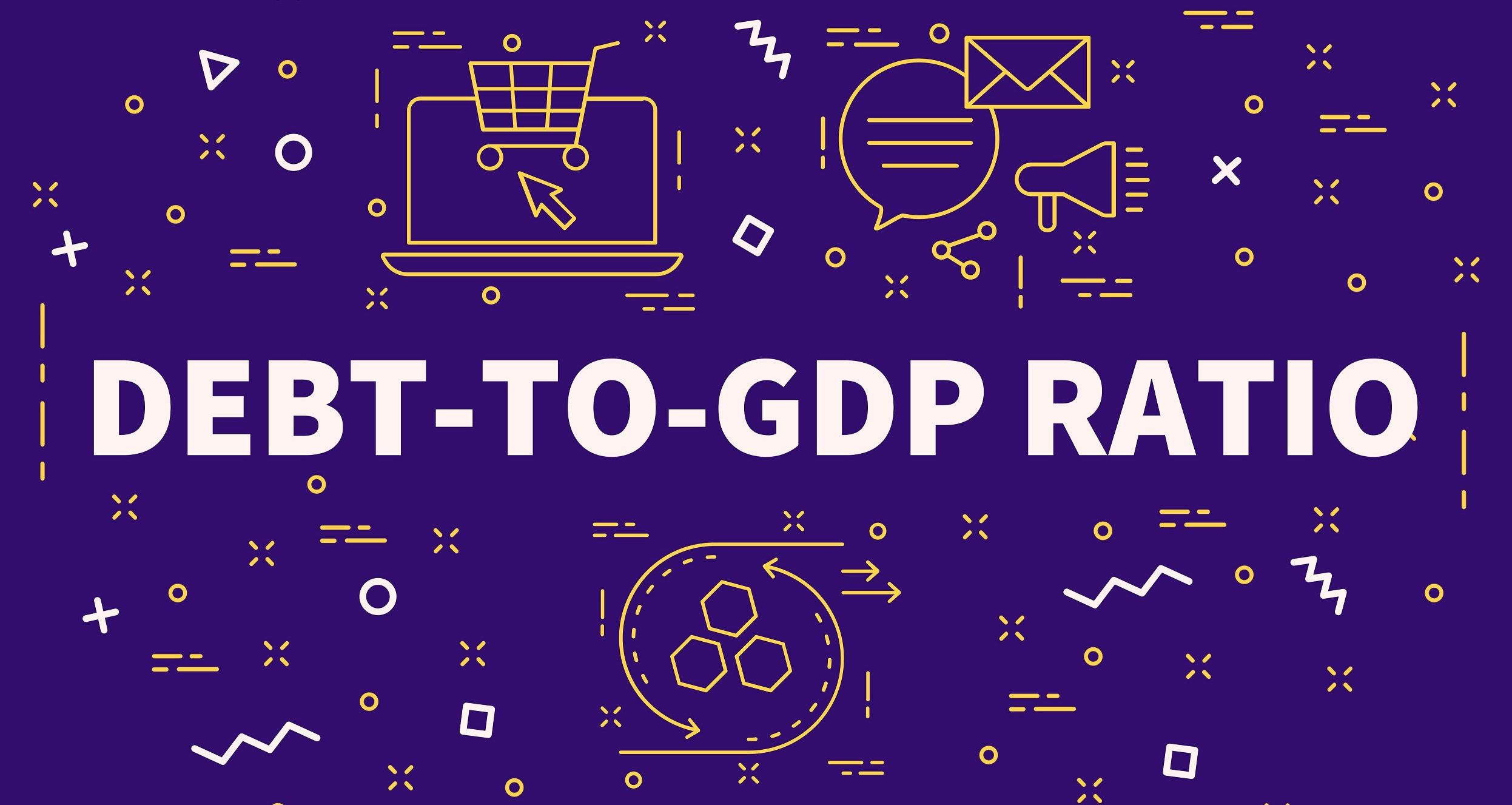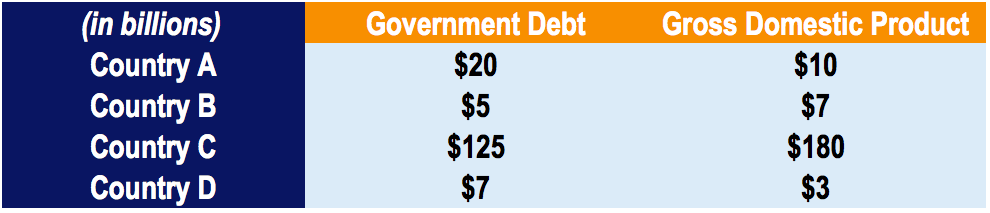# Debt-to-GDP Ratio

A measure of a country's ability to repay its debt

## What is the Debt-to-GDP Ratio?

The debt-to-GDP ratio, commonly used in economics, is the ratio of a country’s debt to its gross domestic product (GDP). Expressed as a percentage, the ratio is used to gauge a country’s ability to repay its debt. In other words, the debt-to-GDP ratio compares a country’s public debt to its annual economic output.### Debt-to-GDP Ratio Formula

The formula for calculating the ratio is as follows:Where:

• Debt is the cumulative amount of a country’s government debt
• Gross Domestic Product is the total value of goods produced and services produced over a given year

### Example of the Debt-to-GDP Ratio

Consider four hypothetical countries with their corresponding national debt and gross domestic product for the year 2020:The debt-to-GDP can be calculated for each country with the formula provided above. The ratio for each country is as follows:

• Country A: \$20 / \$10 = 200.00%
• Country B: \$5 / \$7 = 71.43%
• Country C: \$125 / \$180 = 69.44%
• Country D: \$7 / \$3 = 233.33%

From calculating the debt-to-GDP ratio, we can see that Country A and Country D show the highest ratios. The higher the ratio, the less likely a country will be able to repay its debt. This, in turn, may cause the country to default and cause a financial panic in both the domestic and international markets.

### Interpreting the Debt-To-GDP Ratio

A high debt-to-GDP ratio is undesirable for a country, as a higher ratio indicates a higher risk of default. In a study conducted by the World Bank, a ratio that exceeds 77% for an extended period of time may result in an adverse impact on economic growth. It was indicated that each additional percentage point of debt above that level reduced annual real growth by 1.7%. For reference, the USA’s debt-to-GDP ratio was 105.40% in 2017. Therefore, when the ratio is high (>80%), a country is likely to exhibit a slowdown in economic growth.

The debt-to-GDP ratio is commonly misunderstood, as many think that a ratio exceeding 100% indicates a bankrupt or insolvent country. As indicated below, Japan is a country with a ratio well over 200% for over a decade with no signs of defaulting. Therefore, the ratio does not offer strong insights into a country’s likelihood of default.

### Japan’s Debt-To-GDP Ratio

As indicated above, a high debt-to-GDP ratio is undesirable. However, a high ratio is acceptable if a country is able to pay interest on its debt without refinancing or adversely impacting its economic growth.

For example, Japan’s debt-to-GDP ratio was 253% in 2017 (higher than Greece’s 177% in 2017). Although Japan’s ratio is significantly higher compared to other countries, analysts put the country’s risk of defaulting extremely low. Japan is able to sustain and remain afloat despite a staggering ratio due to the fact that most Japanese government bonds are held by the country’s citizens, resulting in extremely low interest rates.